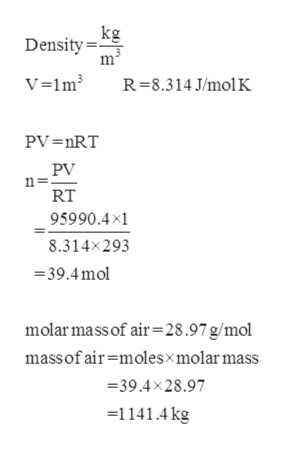# what would be the density of air under 20 C and 720 mmhg? (Hint: has density is proportional to T)

Question
2 views

what would be the density of air under 20 C and 720 mmhg? (Hint: has density is proportional to T)

check_circle

Step 1

Given,

Temperature = 200C = 20+273 = 293K.

Pressure = 720mmHg.

Step 2

The mass and volume of air ...help_outlineImage Transcriptionclosekg Density = m V=lm³ R=8.314 J/mol K PV=nRT PV RT 95990.4×1 8.314x293 = 39.4 mol molar mass of air=28.97g/mol massof air=moles×molar mass =39.4x28.97 =1141.4 kg fullscreen

### Want to see the full answer?

See Solution

#### Want to see this answer and more?

Solutions are written by subject experts who are available 24/7. Questions are typically answered within 1 hour.*

See Solution
*Response times may vary by subject and question.
Tagged in

### Physical Chemistry